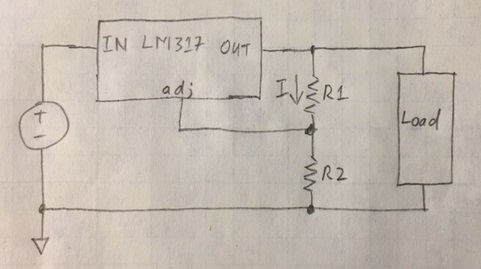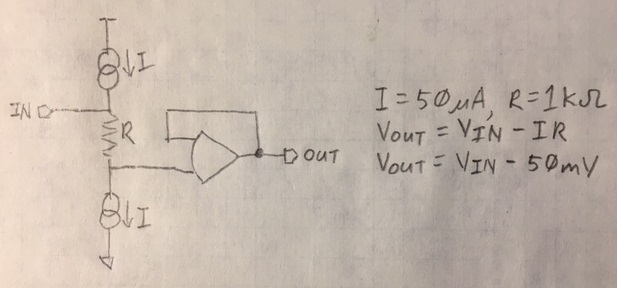## Sunday, November 12, 2017

### A Simple Current Source and An Ode the Resistor

This isn't really a post about using a variable voltage regulator as a current regulator. This is actually a reflection on the lowly resistor's simple but amazing ability to transform a voltage into a current and vice-versa.

Before I get into the wonders of the resistor, I am going to discuss how to make a voltage regulator into a current regulator. I'll use the venerable and classic LM317 as the example regulator but any three terminal variable regulator will work the same wayFigure 1. Voltage Regulator.

It was originally meant to be a voltage regulator, says so right in the name. The LM317 adjusts it's output (by internally adjusting a pass transistor -- pretend it sets a resistor between IN and OUT) until the voltage between ADJ and OUT is 1.25V. It uses negative feedback. Essentially, if you stay inside the drop out voltage and other data sheet limitations, VR1 (the voltage across resistor R1) will be 1.25V.

That makes operation as an adjustable voltage regulator straight forward. The resistor divider formed by R1 and R2 will have 1.25V across its top resistor. You know the math to find the rest.

Of course the resistor sizes set the current through the divider. The current through the divider is VOUT/(R1+R2). Since we know the voltage difference between VOUT and VADJ is 1.25V and this is the voltage across R1, 1.25V/R1 will give the same result. These equations are approximations since they do not include the current that flows into the ADJ terminal. That is a reason to keep the current in the resistors up -- so this error doesn't become relatively important. Many versions of the 317 insist on at least 10mA(!) of output current. If you have to burn some extra current to meet that criteria it might as well be wasted in the resistor divider.

Notice what happened there. The current flowing through R1 keeps flowing through R2 (ignoring a small bit that gets lost into the ADJ pin). That's just Kirchoff's current law. R2 doesn't have anything to do with setting the current. So R2 can be replaced by a circuit that needs to consume that current. An LED is a very common circuit to place there. LEDs have reasonably constant brightness if the current through them is kept constant. This circuit does a decent job of holding the current constant across temperature and voltage.

Did you see what R1 did in that circuit? As long as 1.25V was across it, a constant current flowed through it. It acted as a voltage to current converter. That function is inherent in the definition of a resistor--Ohm's law.

V=IR

The voltage put across the resistor generates a predictable current through the resistor. The resistor acts as a voltage-to-current converter. And it works the other direction.

I=V/R

A current forced through a resistor generates a predictable voltage across the resistor.Figure 3. Current to Voltage Converter.

Here is an example of the resistor being used as a current-to-voltage converter. For this to work well, the two current sources need to be well matched. This is a way to get a small floating voltage. The result is that VOUT is 50mV lower than VIN.Figure 4. Voltage to Current Converter.

Here's an example of the resistor being used as a voltage-to-current converter. The voltage across the resistor is the difference between the power supply and the MOSFET drain (the gate is at the same potential), that is, VDD-VGS. That current gets mirrored over the other MOSFET. Just a different way of thinking about a classic circuit.

There are still aspects of electronics that make my brain smile. The resistor is one of those neat ideas.